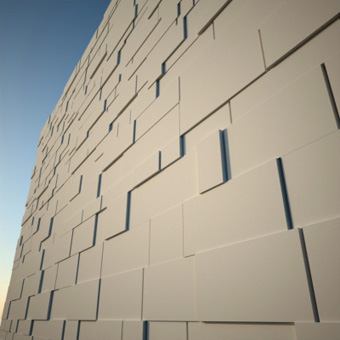# Ali Torabi

Digital Architecture & Technology

## Parametric wall with PARA 2This is a new edition of old video tutorial (Parametric Brick wall) which uses link and math controllers to create a random pattern of stone blocks.

, , , , ,
March 11, 2012 at 10:11 am Comments (0)

## Introducing intersection controller.

In first video you learn how to find the intersection of two coplanar lines. Notice that if two lines are not intersecting program will generates some error. in the second video we find the closest point on one line to a series of lines from another parametric array. Program automatically sets the controllers for point A and B. if two lines are actually intersection the closest point is equal to the intersection point. also you can find the apparent intersection of two lines on a plane. For example the apparent intersection on XY plane is the intersection of projected lines on this plane(intersection from top view) .

, , , , ,
February 28, 2012 at 11:24 am Comments (0)